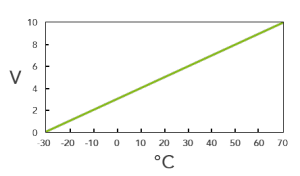# Scaler

## FUNCTIONS

It converts an analogue input value to a different range of values, the scaling is linear.

#### EXAMPLE CORRECTION

Converting a 0-10V analogue signal to a display range of -30 to 70 °C. The paramters need to be set to:

I1 = 0
O1 = -30

I2 = 10
O2 = 70

The linear scaling can be seen in the graph below:## OVERVIEW

#### INPUTS

 AI Analogue Input Connect the analogue value to be corrected here

#### PARAMETER

 I1 Input value 1 Lower limit of the measuring range at the input O1 Output value 1 Lower limit of the corrected range at the output I2 Input value 2 Upper limit of the measuring range at the input O2 Output value 2 Upper limit of the corrected range at the output

#### OUTPUTS

 AQ Analogue output The corrected value is output here